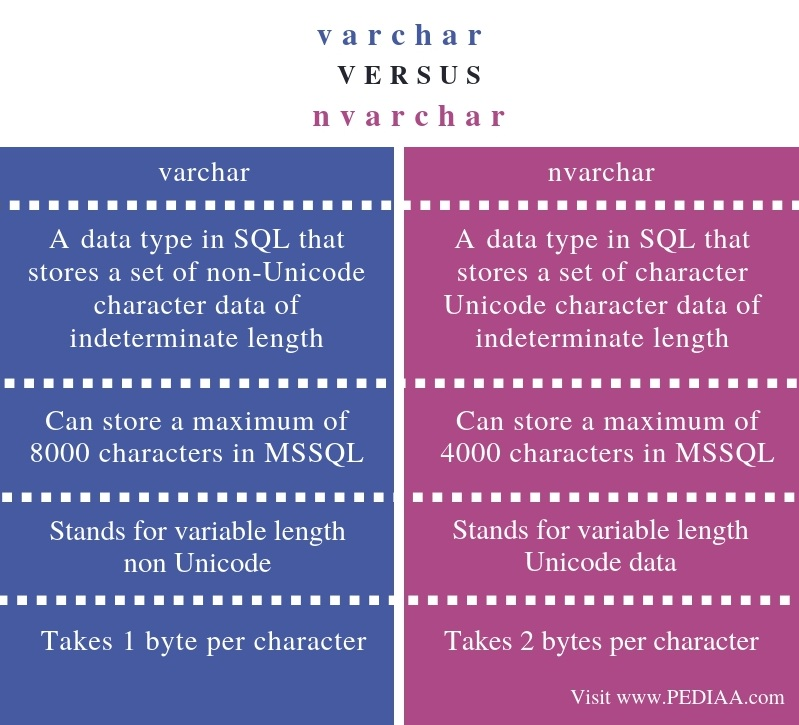# What is the Difference Between varchar and nvarchar

The main difference between varchar and nvarchar is that varchar is a variable length non-Unicode data type while nvarchar is a variable length Unicode data type.

A database is a collection of data, and DBMS is a software that helps to manage databases efficiently. DBMSs such as MSSQL are based on the relational model. They store data in tables, and these tables are connected together. Every table stores a specific set of values. Each row represents a record while the columns represent the attributes. When storing values to the table, each column should have data of a specific type. In other words, each column has a data type. Varchar and nvarchar are two such data types.

### Key Areas Covered

1. What is varchar
– Definition, Functionality
2. What is nvarchar
– Definition, Functionality
3. What is the Difference Between varchar and nvarchar
– Comparison of Key Differences

### Key Terms

Data Type, nvarchar, Unicode, varchar## What is varchar

Structured Query Language (SQL) is the language that helps to retrieve, manage data in DBMS. SQL data types define the type of attributes in tables. Therefore, each column in the DBMS has a specific data type. It describes the type of the data it can store. The programmer can use these data types to create tables.In brief, varchar is a data type that describes a set of character data of indeterminate length. A column of data type varchar can hold letters and numbers. It can hold data up to a certain limit. MSSQL can hold a maximum of 8000 characters. If the programmer uses varchar(max), it can store a maximum length of 2E + 31 characters.

## What is nvarchar

In contrast, nvarchar is a data type that stores variable length Unicode characters. Unicode is an encoding standard that represents letters in languages such as English, Greek, Arabic and various symbols such as mathematical symbols. Therefore, it can represent more characters than varchar. In MSSQL, Each character in nvarchar data type takes 2 bytes.

## Difference Between varchar and nvarchar

### Definition

Varchar is a data type in SQL that stores a set of non-Unicode character data of indeterminate length. In contrast, nvarchar is a data type in SQL that stores a set of character Unicode character data of indeterminate length. Hence, this explains the main difference between varchar and nvarchar.

### Long form

While varchar stands for variable length non Unicode, nvarchar stands for variable-length Unicode data.

### Maximum Length

In MSSQL, varchar can store a maximum of 8000 characters. However, in MSSQL, nvarchar can store a maximum of 4000 characters. Therefore, the storage capacity too is a difference between varchar and nvarchar.

### Number of Bytes

Moreover, another difference between varchar and nvarchar is that the varchar takes 1 byte per character whereas nvacrahr takes 2 bytes per character.

### Conclusion

In summary, varchar and nvarchar are two types of data types in DBMS such as MSSQL. They define what type of data to store in the tables. The difference between varchar and nvarchar is that varchar is a variable length non-unicode data type while nvarchar is a variable length Unicode data type.

##### Reference:

1. “Varchar.” Wikipedia, Wikimedia Foundation, 10 Oct. 2018, Available here.
2. “SQL Data Types.” Www.tutorialspoint.com, Available here.

##### Image Courtesy:

1. “2394312” (CC0) via Pixabay